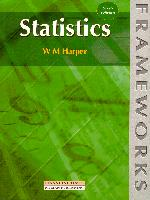Statistics
6th Edition

By W.M. Harper
May 1991
ISBN: 0-273-63426-7507 p.
\$42.50 Paper Original

OUT OF PRINT

This is a comprehensive and concise introduction to the field of statistics. Contents include: nature of statistics, accuracy and approximation, collection of data, tables, graphs, diagrams, preparing a frequency distribution, graphing frequency distributions, fractiles, averages, dispersion and skew, scattergraphs, regression lines, correlation, time series, index numbers, probability, probability distributions and sampling theory.